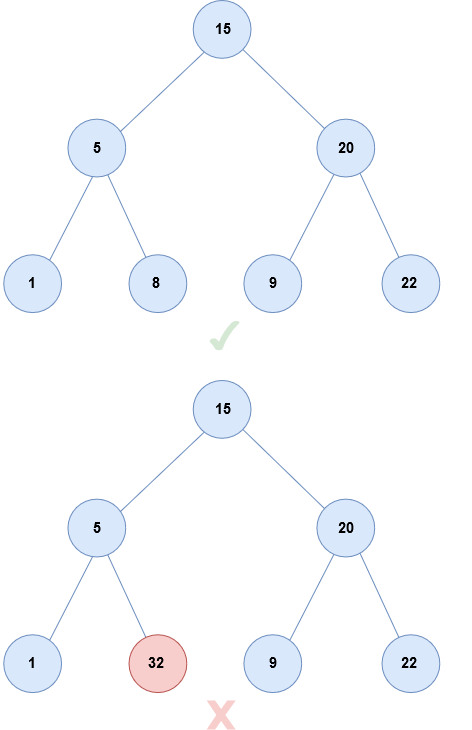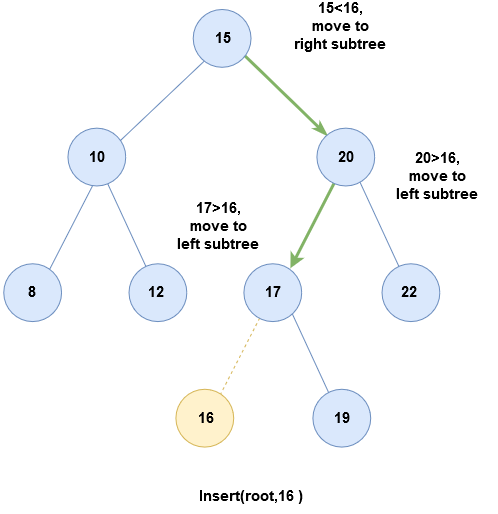# Binary Search Tree

## Binary Search Tree

Binary Search Tree is a special type of binary tree that has a specific order of elements in it. Every node in a binary search tree has a unique value. As it is a binary tree, each tree node has maximum of two children.A Binary Search Tree is used to search a number in `O(log(n))` time.

### Properties of Binary Search Tree

1. All nodes in the left subtree have a lower value than the root node's value.

2. All nodes in the right subtree have a higher value than the root node’s value

3. The left and right subtrees must also be binary search trees i.e. , they must follow the above two properties.

4. Inorder traversal of Binary Search Tree returns a sorted arrangement of its values

## Operations on Binary Search Tree

### Searching

Binary Search Tree has special properties which can be used to efficiently search for an element, unlike in normal Binary Tree where the entire tree needs to be traversed(worst case scenario)
While comparing the value of the item that needs to be searched with the root of the tree, there are three possibilities :

• `val == root→data` item is found, stop the operation.
• `val > root→data` Check only the right subtree since all the values in the left subtree are lesser than `root→data`
• `val < root→data` Check only the left subtree as all values in the right subtree are greater than `root→data`

Performing this operation recursively decreases the search time complexity as only one subtree has to be traversed.

Code

I. Recursive Technique :

``````    node* Search(node* root, int val)
{
if (val == NULL)
return NULL;
if (val == root->data)
return root->data;
if (val < root->data)
return Search(root->left, val);
if (val > root->data)
return Search(root->right, val);
``````

II. Iterative Technique :

``````node* Search(node* node, int val)
{
while(node)
{
if (val == node->data)
return node->data;
if (val < root->data)
node = node->left;
else
node = node->right;
}
return NULL;
}
``````

### Insertion

To insert a node in a Binary Search Tree :

• if root is NULL, create a new node and store value in it.
• else, compare value with data in root node.
• If `val > root→data` , recurse for right subtree
• If `val < root→data`, recurse for left subtree``````node insert(node root, val)
{
if ( root == NULL )
{
root = node(val)
return root
}
if (root->data < val)
root->right = insert(root->right, val)
else if (root->data > val)
root->left = insert(root->left, val)
return root
}
``````
Scroll to Top

### Full Stack Integrated Bootcamp Free Trial

• Please enter a number from 7000000000 to 9999999999.